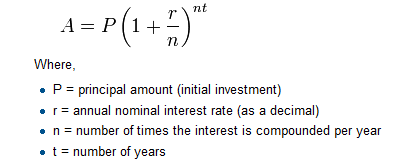# 2.13. Exercises¶

1. Evaluate the following numerical expressions in your head, then use the active code window to check your results:

1. `5 ** 2`

2. `9 * 5`

3. `15 / 12`

4. `12 / 15`

5. `15 // 12`

6. `12 // 15`

7. `5 % 2`

8. `9 % 5`

9. `15 % 12`

10. `12 % 15`

11. `6 % 6`

12. `0 % 7`

1. `5 ** 2  = 25`

2. `9 * 5 = 45`

3. `15 / 12 = 1.25`

4. `12 / 15 = 0.8`

5. `15 // 12 = 1`

6. `12 // 15 = 0`

7. `5 % 2 = 1`

8. `9 % 5 = 4`

9. `15 % 12 = 3`

10. `12 % 15 = 12`

11. `6 % 6 = 0`

12. `0 % 7 = 0`

1. What is the order of the arithmetic operations in the following expression. Evaluate the expression by hand and then check your work.

1. Many people keep time using a 24 hour clock (11 is 11am and 23 is 11pm, 0 is midnight). If it is currently 13 and you set your alarm to go off in 50 hours, it will be 15 (3pm). Write a Python program to solve the general version of the above problem. Ask the user for the time now (in hours), and then ask for the number of hours to wait for the alarm. Your program should output what the time will be on the clock when the alarm goes off.

1. It is possible to name the days 0 through 6 where day 0 is Sunday and day 6 is Saturday. If you go on a wonderful holiday leaving on day number 3 (a Wednesday) and you return home after 10 nights you would return home on a Saturday (day 6) Write a general version of the program which asks for the starting day number, and the length of your stay, and it will tell you the number of day of the week you will return on.

1. Take the sentence: All work and no play makes Jack a dull boy. Store each word in a separate variable, then print out the sentence on one line using `print`.

1. Add parenthesis to the expression `6 * 1 - 2` to change its value from 4 to -6.

1. The formula for computing the final amount if one is earning compound interest is given on Wikipedia asWrite a Python program that assigns the principal amount of 10000 to variable P, assign to n the value 12, and assign to r the interest rate of 8% (0.08). Then have the program prompt the user for the number of years, t, that the money will be compounded for. Calculate and print the final amount after t years.